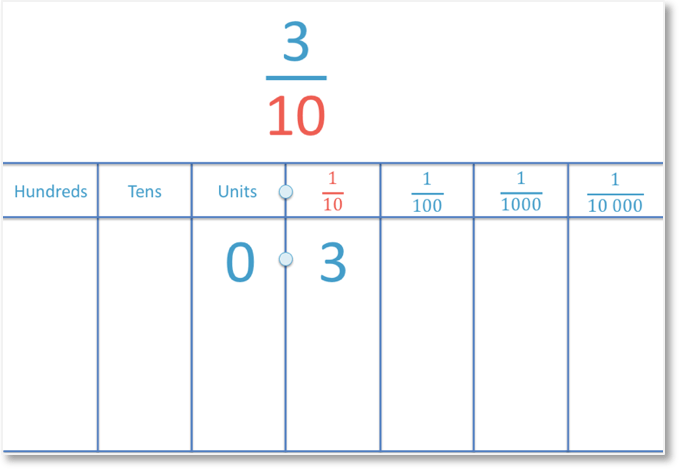# Converting Decimals to Percentages

Converting Decimals to Percentages• The percentage sign is %.
• ‘Per’ means ‘divide’ and ‘cent’ means ‘100’.
• 25% means 25 divided by 100.
• 25 ÷ 100 = 0.25 and so, 25% is the same as 0.25.
• A percentage is simply another way to write a decimal number.
• 0.25 and 25% are the same number.
• To write 0.25 as a percentage, multiply it by 100 and write the % sign afterwards.
• 0.25 × 100 = 25 and so, 0.25 is 25%• To multiply a decimal by 100, simply move each digit two places left while keeping the decimal point still.
• We can see this method above.
• It can be easier to move the decimal point two places right such as in the method below.
• This method may be easier as we only move the one point rather than moving every digit.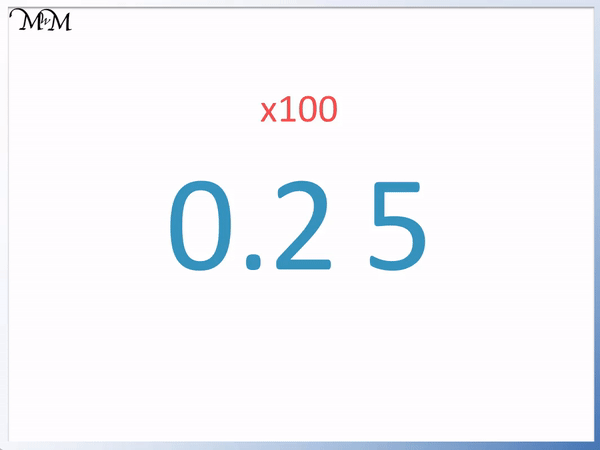To write a decimal as a percentage, multiply it by 100 and write the percentage sign, %.• To write 0.43 as a percentage, we multiply it by 100 and put a % sign afterwards.
• 0.43 × 100 = 43.
• We can work this out by moving the decimal point two places right.
• We write the percentage sign afterwards to show that it is a percentage.
• 0.43 is the same as 43%.
• We can see that we removed the 0 from the front of 0.43 when we multiplied it by 100.
• This is because we don’t write zeros in front of whole numbers.
• We write 43% rather than 043%.Supporting Lessons# Converting Decimals to Percentages

## How to Write a Decimal as a Percent

To write a decimal number as a percentage, multiply it by 100 and then write a % sign afterwards.

To multiply a decimal by 100, use one of the following two methods:

1. Move each digit in the number two places to the left, keeping the decimal point in place.
2. Or alternatively, move the decimal point two places to the right.

Both methods multiply by 100 by multiplying the number by ten and then multiplying it by 10 again.

This is because 10 × 10 = 100.

For example, to write the decimal 0.43 as a percentage, we multiply 0.43 by 100 to get 43 and then write a percentage sign afterwards.

0.43 = 43%We can work out the calculation of 0.43 × 100 using either of the two methods.

In the first method, we keep the decimal point in the same place and move each digit in the number two places to the left.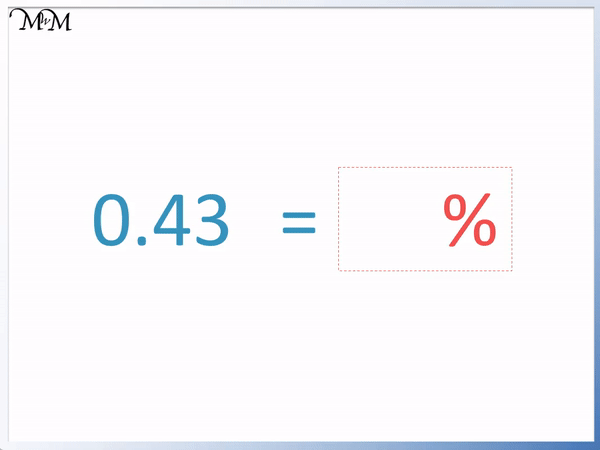We move the 4 into the tens column and the 3 into the units column.

0.43 = 43%

Notice that the 0 at the beginning of 0.43 has been removed because we do not write zeros at the start of whole numbers. We write 43%, not 043%. Otherwise, the digits in 0.43 are in the same order as in 43%.

Moving each digit is a longer process than simply moving the decimal point instead.

When introducing multiplying by 100, it is best to teach it using the method above, as we can see the numbers getting larger.

However, once this process is understood, the most efficient method to teach is the method below, where we only move the decimal point.

Moving the decimal point two places right has the same effect as moving each digit two places left. It is easier to do mentally because we are only moving a single point rather than moving each digit.Again, moving the decimal point two places right, we can see that

0.43 = 43%

We can see that:

A decimal number that has two non-zero decimal digits is written as a percentage by writing both non-zero digits in front of the % sign.

We simply wrote the 4 and the 3 in 0.43 as 43%.

However, it is important to multiply smaller decimals by 100 carefully.

For example, below is the decimal 0.09.

0.09 multiplied by 100 is 9.

0.09 = 9%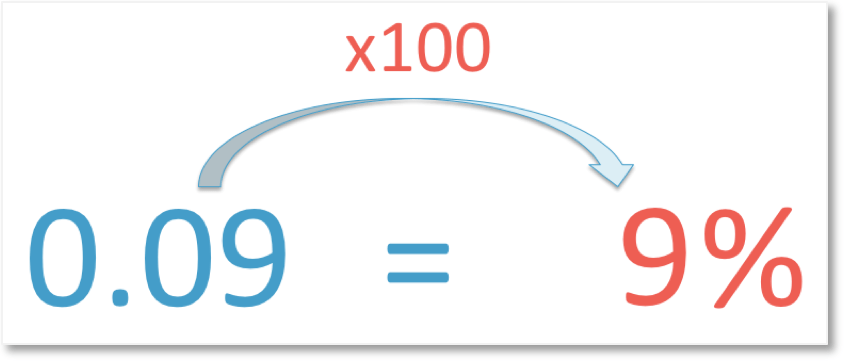Remember to multiply by 100, we move each digit in the number two places left, whilst keeping the decimal point fixed in place.

The 9 moves from the hundredths column into the tenths column and then into the units colum.

We move from 0.09 to 0.9 to 9.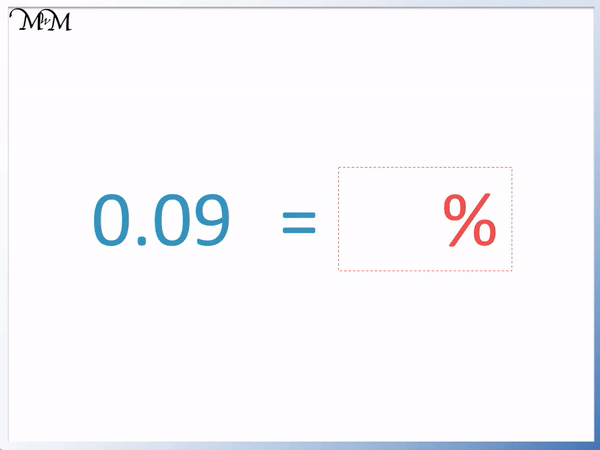0.09 = 9%

We can see that the digit in the hundredths column is the percentage in this example.

Here is another example of converting decimals to percentages.

Here we have 0.2.

To convert a decimal into a percentage, multiply it by 100 and write a percentage sign after it.

The decimal 0.2 written as a percentage is 20%We multiply 0.2 by 100 by moving each digit two places left and keeping the decimal point fixed in place.

The 2 is the only non-zero digit and so, it is the digit we move.

The 2 moves from the tenths column into the units column and then into the tens column.

We go from 0.2 to 2 to 20.We can see that once we move from 0.2 to 2 to 20, we have to put a zero after the 2 to turn it into 20.

To multiply by 100, we multiply by 10 and then 10 again. We move the 2 two places to do this.

## Teaching Converting Decimals to Percentages

When teaching decimals to percentages, it is first important to understand what a percentage is and secondly, to understand how to multiply by 10 and 100.

If these concepts are understood well, then we can use shortcuts to help us multiply by 100.

We can see that 0.25 has a 2 in the tenths column and a 5 in the hundredths column.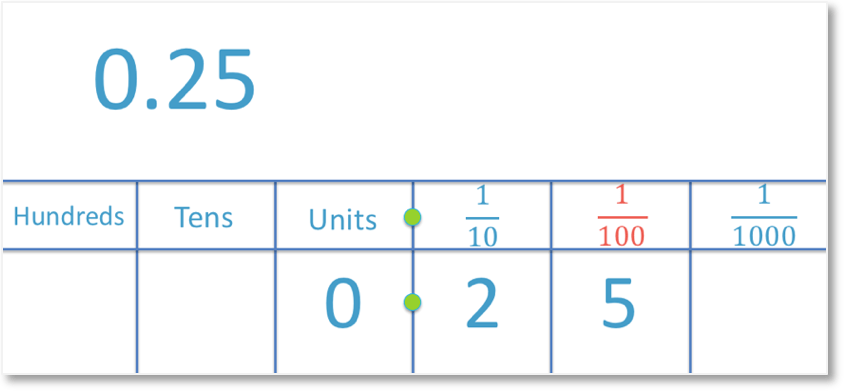The digits to the right of the decimal point represent numbers that are smaller than 1.

0.25 is 25 hundredths because the 5 is in the hundredths column.

0.25 is the same as   25 / 100This fraction is 25 out of 100.

Instead of writing 25 out of 100, we can use the word ‘percent’ to replace ‘out of 100’.

‘Per’ means out of and ‘cent’ means ‘100’.

25 out of 100 can be written as 25 percent and we can even shorten this further by using the percent sign, %, to mean percent.

0.25 is 25 percent, which can be written as 25%.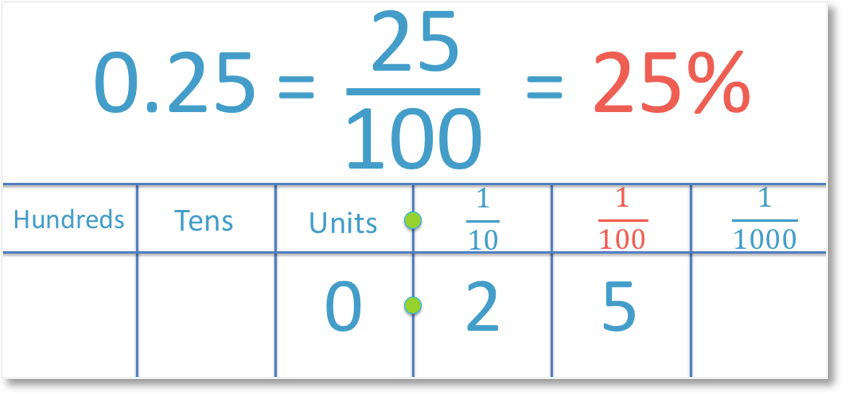25% is said as 25 percent, which means 25 out of 100 parts.It can be helpful to introduce decimals and percentages using a place value grid but it is a slow procedure to do.

We can see that we simply multiply 0.25 by 100 to get the answer of 25%.

Once place value is well understood, we can teach converting decimals to percentages by simply multiplying by 100.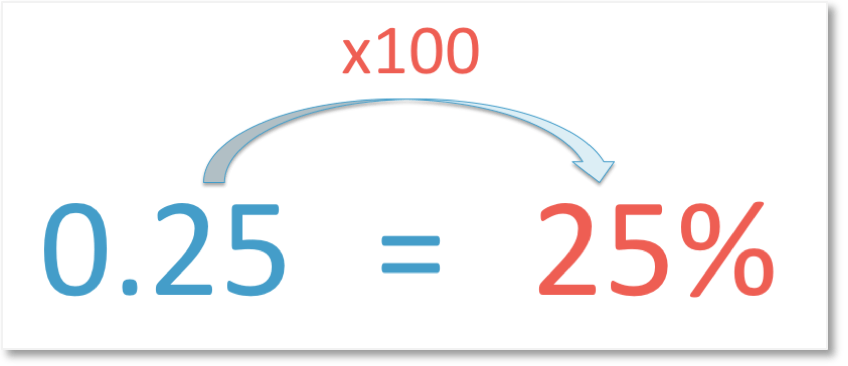We can easily see the comparison between the digits of 2 and 5 in 0.25 and 25%.When first teaching multiplying by 100, we show the digits getting larger on a place value grid.

When multiplying by 100, the digits get larger and the decimal point does not actually move.

100 is the same as 10 × 10.

To multiply by 100, we multiply by 10 and then multiply by 10 again.

Each time we multiply by 10, we move each digit one place left on the place value grid.

To multiply by 100, move each non-zero digit separately two places to the left.0.25 becomes 2.5 when we multiply it by 10 and then it becomes 25 when we multiply by 10 again.

However, we can see that it can be quicker to move the decimal point two places right instead of moving every digit two places left.

Whilst the decimal point does not actually ever move, this is a trick that we can use as it will give us the correct answer.

It is easier to move the decimal point rather than every digit because we only need to move one point. This makes this method the quickest and easiest method to use as well as being the best mental method to use.Now try our lesson on Converting Fractions to Decimals where we learn how to write a fraction as a decimal.